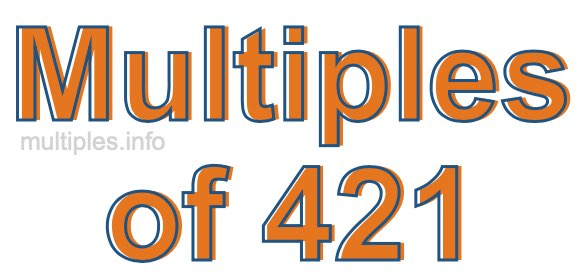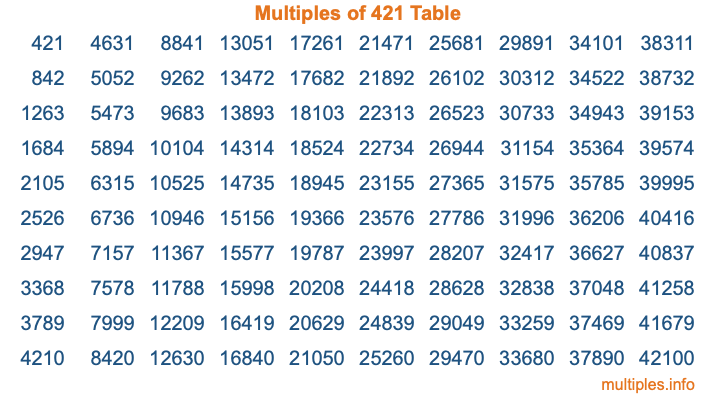Multiples of 421Welcome to the Multiples of 421 page. Here we will first teach you everything you will ever need to know about the multiples of 421, and then give you a study guide summary of everything we taught you to make sure you remember it all. Use this page to look up facts and learn information about the multiples of 421. This page will make you a multiples of four hundred twenty-one expert!

Definition of Multiples of 421
Multiples of 421 are all the numbers that when divided by 421 equal an integer. Each of the multiples of 421 are called a multiple. A multiple of 421 is created by multiplying 421 by an integer.

Therefore, to create a list of multiples of 421, you start with 1 multiplied by 421, then 2 multiplied by 421, then 3 multiplied by 421, and so on for as long as you want. Thus, the list of the first five multiples of 421 is 421, 842, 1263, 1684, and 2105. To see a larger list of multiples of 421, see the printable image of Multiples of 421 further down on this page. We also have a category where you can choose any nth multiple of 421.

Multiples of 421 Checker
The Multiples of 421 Checker below checks to see if any number of your choice is a multiple of 421. In other words, it checks to see if there is any number (integer) that when multiplied by 421 will equal your number. To do that, we divide your number by 421. If the the quotient is an integer, then your number is a multiple of 421.

Is  a multiple of 421?

Least Common Multiple of 421 and ...
A Least Common Multiple (LCM) is the lowest multiple that two or more numbers have in common. This is also called the smallest common multiple or lowest common multiple and is useful to know when you are adding our subtracting fractions. Enter one or more numbers below (421 is already entered) to find the LCM.

Check out our LCM Calculator if you need more details about the Least Common Multiple or if you need the LCM for different numbers for adding and subtraction fractions.

nth Multiple of 421
As we stated above, 421 is the first multiple of 421, 842 is the second multiple of 421, 1263 is the third multiple of 421, and so on. Enter a number below to find the nth multiple of 421.

th multiple of 421

Multiples of 421 vs Factors of 421
421 is a multiple of 421 and a factor of 421, but that is where the similarities end. All postive multiples of 421 are 421 or greater than 421. All positive factors of 421 are 421 or less than 421.

Below is the beginning list of multiples of 421 and the factors of 421 so you can compare:

Multiples of 421: 421, 842, 1263, 1684, 2105, etc.

Factors of 421: 1, 421

As you can see, the multiples of 421 are all the numbers that you can divide by 421 to get a whole number. The factors of 421, on the other hand, are all the whole numbers that you can multiply by another whole number to get 421.

It's also interesting to note that if a number (x) is a factor of 421, then 421 will also be a multiple of that number (x).

Multiples of 421 vs Divisors of 421
The divisors of 421 are all the integers that 421 can be divided by evenly. Below is a list of the divisors of 421.

Divisors of 421: 1, 421

The interesting thing to note here is that if you take any multiple of 421 and divide it by a divisor of 421, you will see that the quotient is an integer.

Multiples of 421 Table
Below is an image of the first 100 multiples of 421 in a table. The table is in chronological order, column by column. The first column has the first ten multiples of 421, the second column has the next ten multiples of 421, and so on.The Multiples of 421 Table is also referred to as the 421 Times Table or Times Table of 421. You are welcome to print out our table for your studies.

Negative Multiples of 421
Although not often discussed or needed in math, it is worth mentioning that you can make a list of negative multiples of 421 by multiplying 421 by -1, then by -2, then by -3, and so on, to get the following list of negative multiples of 421:

-421, -842, -1263, -1684, -2105, etc.

Multiples of 421 Summary
Below is a summary of important Multiples of 421 facts that we have discussed on this page. To retain the knowledge on this page, we recommend that you read through the summary and explain to yourself or a study partner why they hold true.

There are an infinite number of multiples of 421.

A multiple of 421 divided by 421 will equal a whole number.

421 divided by a factor of 421 equals a divisor of 421.

The nth multiple of 421 is n times 421.

The largest factor of 421 is equal to the first positive multiple of 421.

421 is a multiple of every factor of 421.

421 is a multiple of 421.

A multiple of 421 divided by a divisor of 421 equals an integer.

421 divided by a divisor of 421 equals a factor of 421.

Any integer times 421 will equal a multiple of 421.

Multiples of a Number
Here you can get the multiples of another number, all with the same attention to detail as we did for multiples of 421 on this page.

Multiples of
Multiples of 422
Did you find our page about multiples of four hundred twenty-one educational? Do you want more knowledge? Check out the multiples of the next number on our list!